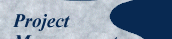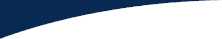## Appendix

Bayesian inference is a method of statistical inference in which Bayes' Theorem is used to update probability based on new information. Although the theorem is simple and elegant — everything is contained in only one equation — its daily application spawns lots of auxiliary paraphernalia, significantly obscuring matters.

We eliminate this problem with a nomogram — a graphical calculator — encapsulating all the mathematical apparatus. Nomograms are difficult to construct but extremely easy to use, allowing us to solve equations without doing any algebra. We'd like a nomogram to take us from our pretest probability to the posttest probability with minimum effort.

For a positive test result, the factor that governs the transformation is LR+, and for a negative test result, LR-. Because we start with e and f from our 2x2 historical matrix, we need a calculator taking us from the pair (e, f) to the pair (LR+, LR-).

We constructed that nomogram in a previous work, in which we used Bayes' Theorem in medical diagnosis. That problem is analogous to ours, with the pretest probability representing the original probability of having a disease, the likelihood ratios LR+ and LR- describing the power of the binary diagnostic test, and the posttest probability representing the revised probability of having the disease. However, instead of e and f, medical diagnostic tests are characterized by two other parameters, so we needed to transform the pair (sensitivity, specificity) to (LR+, LR-).

We accomplished all these objectives with one nomogram with multiple scales. We reproduce this nomogram below, marked up with the four lines that give us the solution to our problem. Ignore the scales for sensitivity and specificity, as they don't apply here.